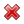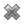# Fight Finance

#### CoursesTagsRandomAllRecentScoresScoreskeithphw $6,001.61 Jade$1,815.80 Chu $789.98 royal ne...$750.00 Leehy $713.33 JennyLI$625.61 Visitor $570.00 Visitor$550.00 Visitor $550.00 ZOE HY$540.00 Visitor $540.00 Yizhou$489.18 Visitor $464.70 Jasper.sun$460.00 Visitor $460.00 Visitor$460.00 Visitor $442.43 Visitor$380.00 Visitor $380.00 niki,zhang$380.00

Question 906  effective rate, return types, net discrete return, return distribution, price gains and returns over time

For an asset's price to double from say $1 to$2 in one year, what must its effective annual return be? Note that an effective annual return is also called a net discrete return per annum. If the price now is $P_0$ and the price in one year is $P_1$ then the effective annul return over the next year is:

$$r_\text{effective annual} = \dfrac{P_1 - P_0}{P_0} = \text{NDR}_\text{annual}$$